# actors¶

Submodule extending the vtkActor, vtkVolume and vtkImageActor objects functionality.

## Actor¶

class vtkplotter.actors.Actor(inputobj=None, c=None, alpha=1, computeNormals=False)[source]

Bases: vtkRenderingCorePython.vtkFollower, vtkplotter.actors.Prop

Build an instance of object Actor derived from vtkActor.

Input can be vtkPolyData, vtkActor, or a python list of [vertices, faces].

If input is any of vtkUnstructuredGrid, vtkStructuredGrid or vtkRectilinearGrid the goemetry is extracted. In this case the original VTK data structures can be accessed with: actor.inputdata().

Finally input can be a list of vertices and their connectivity (faces of the polygonal mesh). For point clouds - e.i. no faces - just substitute the faces list with None.

E.g.: Actor( [ [[x1,y1,z1],[x2,y2,z2], …], [[0,1,2], [1,2,3], …] ] )

Parameters: c – color in RGB format, hex, symbol or name alpha (float) – opacity value wire (bool) – show surface as wireframe bc – backface color of internal surface texture (str) – jpg file name or surface texture name computeNormals (bool) – compute point and cell normals at creation

Hint

A mesh can be built from vertices and their connectivity. See e.g.:

addCurvatureScalars(method=0, lut=None)[source]

Add scalars to Actor that contains the curvature calculated in three different ways.

Parameters: method (int) – 0-gaussian, 1-mean, 2-max, 3-min curvature. lut – optional vtkLookUpTable up table. from vtkplotter import Torus Torus().addCurvatureScalars().show()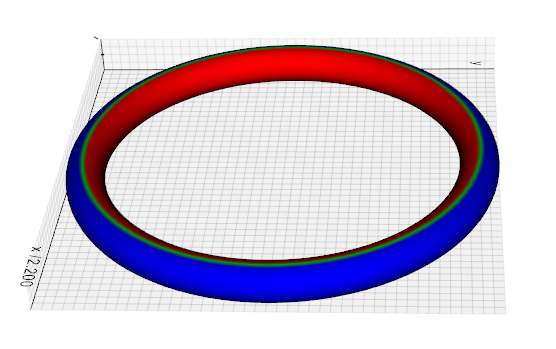addElevationScalars(lowPoint=(), highPoint=(), vrange=(), lut=None)[source]

Add to Actor a scalar array that contains distance along a specified direction.

Parameters: low (list) – one end of the line (small scalar values). Default (0,0,0). high (list) – other end of the line (large scalar values). Default (0,0,1). vrange (list) – set the range of the scalar. Default is (0, 1). lut – optional vtkLookUpTable up table (see makeLUT method). from vtkplotter import Sphere s = Sphere().addElevationScalars(lowPoint=(0,0,0), highPoint=(1,1,1)) s.addScalarBar().show(axes=1) |elevation| 
addGaussNoise(sigma)[source]

Parameters: sigma (float) – sigma is expressed in percent of the diagonal size of actor. from vtkplotter import Sphere Sphere().addGaussNoise(1.0).show() 
addIDs(asfield=False)[source]

Generate point and cell ids.

Parameters: asfield (bool) – flag to control whether to generate scalar or field data.
addPointVectors(vectors, name)[source]

Add a point vector field to the actor’s polydata assigning it a name.

addScalarBar(pos=(0.8, 0.05), title='', titleXOffset=0, titleYOffset=15, titleFontSize=12, nlabels=10, c=None, horizontal=False, vmin=None, vmax=None)[source]

Add a 2D scalar bar to actor.

addScalarBar3D(pos=(0, 0, 0), normal=(0, 0, 1), sx=0.1, sy=2, title='', titleXOffset=-1.4, titleYOffset=0.0, titleSize=1.5, titleRotation=0.0, nlabels=9, precision=3, labelOffset=0.4, c=None, alpha=1, cmap=None)[source]

Draw a 3D scalar bar to actor.

alpha(opacity=None)[source]

Set/get actor’s transparency. Same as actor.opacity().

area(value=None)[source]

Get/set the surface area of actor.

averageSize()[source]

Calculate the average size of a mesh. This is the mean of the vertex distances from the center of mass.

backColor(bc=None)[source]

Set/get actor’s backface color.

backFaceCulling(value=True)[source]

Set culling of polygons based on orientation of normal with respect to camera.

bc(backColor=False)[source]

Shortcut for actor.backColor().

boundaries(boundaryEdges=True, featureAngle=65, nonManifoldEdges=True)[source]

Return an Actor that shows the boundary lines of an input mesh.

Parameters: boundaryEdges (bool) – Turn on/off the extraction of boundary edges. featureAngle (float) – Specify the feature angle for extracting feature edges. nonManifoldEdges (bool) – Turn on/off the extraction of non-manifold edges.
cap(returnCap=False)[source]

Generate a “cap” on a clipped actor, or caps sharp edges.

cellCenters()[source]

Get the list of cell centers of the mesh surface.

cellColors(scalars_or_colors, cmap='jet', alpha=1, alphaPerCell=False, mode='scalars', bands=None, vmin=None, vmax=None)[source]

Set individual cell colors by setting a list of scalars.

If mode='scalars' (default), set individual cell colors by the provided list of scalars.

If mode='colors', colorize the faces of a mesh one by one, passing a 1-to-1 list of colors and optionally a list of transparencies.

Parameters: alpha (float, list) – mesh transparency. Can be a list of values one for each cell.

Only relevant with mode='scalars' (default):

Parameters: cmap (str, list, vtkLookupTable, matplotlib.colors.LinearSegmentedColormap) – color map scheme to transform a real number into a color. bands (int) – group scalars in this number of bins, typically to form bands of stripes. vmin (float) – clip scalars to this minimum value vmax (float) – clip scalars to this maximum value

Only relevant with mode='colors':

Parameters: alphaPerCell (bool) – Only matters if alpha is a sequence. If so: if True assume that the list of opacities is independent on the colors (same color cells can have different opacity), this can be very slow for large meshes, if False [default] assume that the alpha matches the color list (same color has the same opacity). This is very fast even for large meshes.
centerOfMass()[source]

Get the center of mass of actor.

clean(tol=None)[source]

Clean actor’s polydata. Can also be used to decimate a mesh if tol is large. If tol=None only removes coincident points.

Parameters: tol – defines how far should be the points from each other in terms of fraction of the bounding box length.
clone(transformed=True)[source]

Clone a Actor(vtkActor) and make an exact copy of it.

Parameters: transformed – if False ignore any previous transformation applied to the mesh.
closestPoint(pt, N=1, radius=None, returnIds=False)[source]

Find the closest point(s) on a mesh given from the input point pt.

Parameters: N (int) – if greater than 1, return a list of N ordered closest points. radius (float) – if given, get all points within that radius. returnIds (bool) – return points IDs instead of point coordinates.

Note

The appropriate kd-tree search locator is built on the fly and cached for speed.

color(c=False)[source]

Set/get actor’s color. If None is passed as input, will use colors from active scalars. Same as c().

computeNormals(points=True, cells=True)[source]

Compute cell and vertex normals for the actor’s mesh.

Warning

Mesh gets modified, output can have a different nr. of vertices.

connectedCells(index, returnIds=False)[source]

Find all cellls connected to an input vertex specified by its index.

connectedVertices(index, returnIds=False)[source]

Find all vertices connected to an input vertex specified by its index.

Parameters: returnIds (bool) – return vertex IDs instead of vertex coordinates.
coordinates(transformed=True, copy=False)[source]

Return the list of vertex coordinates of the input mesh. Same as actor.getPoints().

Parameters: transformed (bool) – if False ignore any previous transformation applied to the mesh. copy (bool) – if False return the reference to the points so that they can be modified in place, otherwise a copy is built.
crop(top=None, bottom=None, right=None, left=None, front=None, back=None)[source]

Crop an Actor object. Input object is modified.

Parameters: top (float) – fraction to crop from the top plane (positive z) bottom (float) – fraction to crop from the bottom plane (negative z) front (float) – fraction to crop from the front plane (positive y) back (float) – fraction to crop from the back plane (negative y) right (float) – fraction to crop from the right plane (positive x) left (float) – fraction to crop from the left plane (negative x)

Example

from vtkplotter import Sphere
Sphere().crop(right=0.3, left=0.1).show()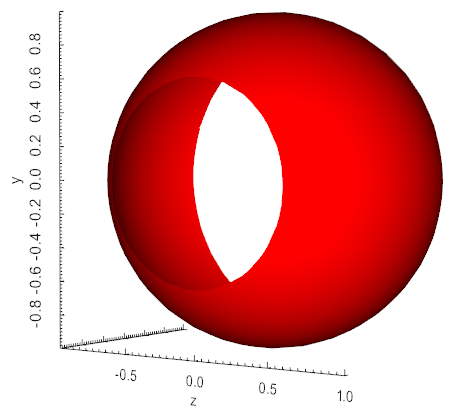cutWithMesh(mesh, invert=False)[source]

Cut an Actor mesh with another vtkPolyData or Actor.

Parameters: invert (bool) – if True return cut off part of actor.
cutWithPlane(origin=(0, 0, 0), normal=(1, 0, 0), showcut=False)[source]

Takes a vtkActor and cuts it with the plane defined by a point and a normal.

Parameters: origin – the cutting plane goes through this point normal – normal of the cutting plane showcut – if True show the cut off part of the mesh as thin wireframe. from vtkplotter import Cube cube = Cube().cutWithPlane(normal=(1,1,1)) cube.bc('pink').show()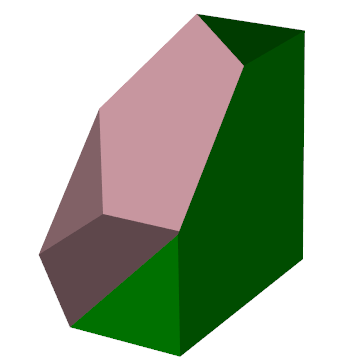cutWithPointLoop(points, invert=False)[source]

Cut an Actor mesh with a set of points forming a closed loop.

decimate(fraction=0.5, N=None, method='quadric', boundaries=False)[source]

Downsample the number of vertices in a mesh to fraction.

Parameters: fraction (float) – the desired target of reduction. N (int) – the desired number of final points (fraction is recalculated based on it). method (str) – can be either ‘quadric’ or ‘pro’. In the first case triagulation will look like more regular, irrespective of the mesh origianl curvature. In the second case triangles are more irregular but mesh is more precise on more curved regions. boundaries (bool) – (True), in pro mode decide whether to leave boundaries untouched or not.

Note

Setting fraction=0.1 leaves 10% of the original nr of vertices.

deletePoints(indices)[source]

Delete a list of vertices identified by their index.

diagonalSize()[source]

Get the length of the diagonal of actor bounding box.

distanceToMesh(actor, signed=False, negate=False)[source]

Computes the (signed) distance from one mesh to another.

faces()[source]

Get cell connettivity ids as a python list. The output format is: [[id0 … idn], [id0 … idm], etc].

Same as getConnectivity().

fillHoles(size=None)[source]

Identifies and fills holes in input mesh. Holes are identified by locating boundary edges, linking them together into loops, and then triangulating the resulting loops.

Parameters: size (float) – approximate limit to the size of the hole that can be filled.

Example: fillholes.py

findCellsWithin(xbounds=(), ybounds=(), zbounds=(), c=None)[source]

Find cells that are within specified bounds. Setting a color will add a vtk array to colorize these cells.

flat()[source]

Set surface interpolation to Flat.flipNormals()[source]

Flip all mesh normals. Same as actor.mirror(‘n’).

followCamera(cam=None)[source]

Actor object will follow camera movements and stay locked to it.

Parameters: cam (vtkCamera) – if None the text will auto-orient itself to the active camera. A vtkCamera object can also be passed.
frontFaceCulling(value=True)[source]

Set culling of polygons based on orientation of normal with respect to camera.

getConnectivity()[source]
Get cell connettivity ids as a python list. The format is:
[[id0 … idn], [id0 … idm], etc].

Same as faces().

getPoint(i)[source]

Retrieve specific i-th point coordinates in mesh. Actor transformation is reset to its mesh position/orientation.

Parameters: i (int) – index of vertex point.

Warning

if used in a loop this can slow down the execution by a lot.

actor.getPoints()

getPoints(transformed=True, copy=False)[source]

Return the list of vertex coordinates of the input mesh. Same as actor.coordinates().

Parameters: transformed (bool) – if False ignore any previous transformation applied to the mesh. copy (bool) – if False return the reference to the points so that they can be modified in place.
getPolygons()[source]

Get cell connettivity ids as a 1D array. The vtk format is: [nids1, id0 … idn, niids2, id0 … idm, etc].

gouraud()[source]

Set surface interpolation to Gouraud.

insidePoints(points, invert=False, tol=1e-05)[source]

Return the sublist of points that are inside a polydata closed surface.

intersectWithLine(p0, p1)[source]

Return the list of points intersecting the actor along the segment defined by two points p0 and p1.

Example: from vtkplotter import * s = Spring(alpha=0.2) pts = s.intersectWithLine([0,0,0], [1,0.1,0]) ln = Line([0,0,0], [1,0.1,0], c='blue') ps = Points(pts, r=10, c='r') show(s, ln, ps, bg='white')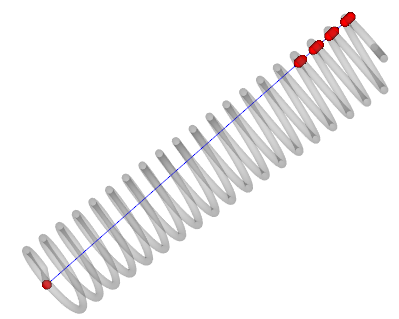isInside(point, tol=0.0001)[source]

Return True if point is inside a polydata closed surface.

isolines(n=10, vmin=None, vmax=None)[source]

Return a new Actor representing the isolines of the active scalars.

Parameters: n (int) – number of isolines in the range vmin (float) – minimum of the range vmax (float) – maximum of the range
lc(lineColor=None)[source]

Set/get color of mesh edges. Same as lineColor().

lineColor(lc=None)[source]

Set/get color of mesh edges. Same as lc().

lineWidth(lw=None)[source]

Set/get width of mesh edges. Same as lw().

lw(lineWidth=None)[source]

Set/get width of mesh edges. Same as lineWidth().

maxBoundSize()[source]

Get the maximum dimension in x, y or z of the actor bounding box.

mirror(axis='x')[source]

Mirror the actor polydata along one of the cartesian axes.

Note

axis='n', will flip only mesh normals.

normalAt(i)[source]

Return the normal vector at vertex point i.

normalize()[source]

Shift actor’s center of mass at origin and scale its average size to unit.

normals(cells=False)[source]

Retrieve vertex normals as a numpy array.

Params bool cells:
if True return cell normals.
opacity(alpha=None)[source]

Set/get actor’s transparency. Same as actor.alpha().

phong()[source]

Set surface interpolation to Phong.

pointColors(scalars_or_colors, cmap='jet', alpha=1, mode='scalars', bands=None, vmin=None, vmax=None)[source]

Set individual point colors by providing a list of scalar values and a color map. scalars can be a string name of the vtkArray.

if mode='colors', colorize vertices of a mesh one by one, passing a 1-to-1 list of colors.

Parameters: alphas (list) – single value or list of transparencies for each vertex cmap (str, list, vtkLookupTable, matplotlib.colors.LinearSegmentedColormap) – color map scheme to transform a real number into a color. alpha (float, list) – mesh transparency. Can be a list of values one for each vertex. bands (int) – group scalars in this number of bins, typically to form bands or stripes. vmin (float) – clip scalars to this minimum value vmax (float) – clip scalars to this maximum value
pointSize(ps=None)[source]

Set/get actor’s point size of vertices. Same as ps()

polydata(transformed=True)[source]

Returns the vtkPolyData object of an Actor.

Note

If transformed=True returns a copy of polydata that corresponds to the current actor’s position in space.

projectOnPlane(direction='z')[source]

Project the mesh on one of the Cartesian planes.

ps(pointSize=None)[source]

Set/get actor’s point size of vertices. Same as pointSize()

quantize(binSize)[source]

The user should input binSize and all {x,y,z} coordinates will be quantized to that absolute grain size.

Example

from vtkplotter import Paraboloid
Paraboloid().lw(0.1).quantize(0.1).show()

reverse(cells=True, normals=False)[source]

Reverse the order of polygonal cells and/or reverse the direction of point and cell normals. Two flags are used to control these operations:

• cells=True reverses the order of the indices in the cell connectivity list.
• normals=True reverses the normals by multiplying the normal vector by -1
(both point and cell normals, if present).
scalars(name_or_idx=None, datatype='point')[source]

Retrieve point or cell scalars using array name or index number, and set it as the active one.

If no input is given return the list of names of existing arrays.

Parameters: datatype (str) – search given name in point-data or cell-data
setPoint(i, p)[source]

Set specific i-th point coordinates in mesh. Actor transformation is reset to its original mesh position/orientation.

Parameters: i (int) – index of vertex point. p (list) – new coordinates of mesh point.

Warning

if used in a loop this can slow down the execution by a lot.

actor.Points()

setPoints(pts)[source]

Set specific points coordinates in mesh. Input is a python list. Actor transformation is reset to its mesh position/orientation.

Parameters: pts (list) – new coordinates of mesh vertices.
shrink(fraction=0.85)[source]

Shrink the triangle polydata in the representation of the input mesh.

Example

from vtkplotter import *
s = Sphere(r=0.2).pos(0,0,-0.5)
show(pot, s)

silhouette(direction=None, borderEdges=True, featureAngle=None)[source]

Return a new line Actor which corresponds to the outer silhouette of the input as seen along a specified direction, this can also be a vtkCamera object.

Parameters: direction (list) – viewpoint direction vector. If None this is guessed by looking at the minimum of the sides of the bounding box. borderEdges (float) – enable or disable generation of border edges borderEdges – minimal angle for sharp edges detection. If set to False the functionality is disabled.
smoothLaplacian(niter=15, relaxfact=0.1, edgeAngle=15, featureAngle=60)[source]

Adjust mesh point positions using Laplacian smoothing.

Parameters: niter (int) – number of iterations. relaxfact (float) – relaxation factor. Small relaxfact and large niter are more stable. edgeAngle (float) – edge angle to control smoothing along edges (either interior or boundary). featureAngle (float) – specifies the feature angle for sharp edge identification.
smoothWSinc(niter=15, passBand=0.1, edgeAngle=15, featureAngle=60)[source]

Adjust mesh point positions using the Windowed Sinc function interpolation kernel.

Parameters: niter (int) – number of iterations. passBand (float) – set the passband value for the windowed sinc filter. edgeAngle (float) – edge angle to control smoothing along edges (either interior or boundary). featureAngle (float) – specifies the feature angle for sharp edge identification.
stretch(q1, q2)[source]

Stretch actor between points q1 and q2. Mesh is not affected.

Note

for Actors like helices, Line, cylinders, cones etc., two attributes actor.base, and actor.top are already defined.

subdivide(N=1, method=0)[source]

Increase the number of vertices of a surface mesh.

Parameters: N (int) – number of subdivisions. method (int) – Loop(0), Linear(1), Adaptive(2), Butterfly(3)

Hint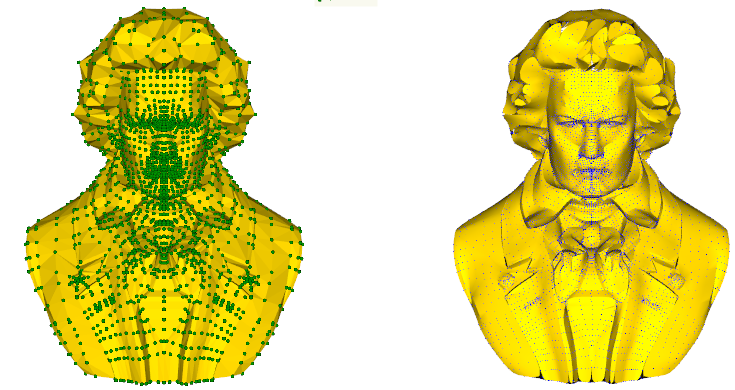tutorial.py

texture(tname, tcoords=None, interpolate=True, repeat=True, edgeClamp=False)[source]

Assign a texture to actor from image file or predefined texture tname. If tname is None texture is disabled.

Parameters: interpolate (bool) – turn on/off linear interpolation of the texture map when rendering. repeat (bool) – repeat of the texture when tcoords extend beyond the [0,1] range. edgeClamp (bool) – turn on/off the clamping of the texture map when the texture coords extend beyond the [0,1] range. Only used when repeat is False, and edge clamping is supported by the graphics card.
threshold(scalars, vmin=None, vmax=None, useCells=False)[source]

Extracts cells where scalar value satisfies threshold criterion.

Parameters: scalars (str, list) – name of the scalars array. vmin (float) – minimum value of the scalar vmax (float) – maximum value of the scalar useCells (bool) – if True, assume array scalars refers to cells.
transformMesh(transformation)[source]

Apply this transformation to the polygonal mesh, not to the actor’s transformation, which is reset.

Parameters: transformation – vtkTransform or vtkMatrix4x4 object.
triangle(verts=True, lines=True)[source]

Converts actor polygons and strips to triangles.

Parameters: verts (bool) – if True, break input vertex cells into individual vertex cells (one point per cell). If False, the input vertex cells will be ignored. lines (bool) – if True, break input polylines into line segments. If False, input lines will be ignored and the output will have no lines.
updateMesh(polydata)[source]

Overwrite the polygonal mesh of the actor with a new one.

volume(value=None)[source]

Get/set the volume occupied by actor.

wireframe(value=True)[source]

Set actor’s representation as wireframe or solid surface. Same as wireframe().

write(filename='mesh.vtk', binary=True)[source]

Write actor’s polydata in its current transformation to file.

xbounds()[source]

Get the actor bounds [xmin,xmax].

ybounds()[source]

Get the actor bounds [ymin,ymax].

zbounds()[source]

Get the actor bounds [zmin,zmax].

## Assembly¶

class vtkplotter.actors.Assembly(actors)[source]

Bases: vtkRenderingCorePython.vtkAssembly, vtkplotter.actors.Prop

Group many actors as a single new actor as a vtkAssembly.

diagonalSize()[source]

Return the maximum diagonal size of the Actors of the Assembly.

getActor(i)[source]

Get i-th vtkActor object from a vtkAssembly.

getActors()[source]

Unpack a list of vtkActor objects from a vtkAssembly.

lighting(style='', ambient=None, diffuse=None, specular=None, specularPower=None, specularColor=None, enabled=True)[source]

Set the lighting type to all Actor in the Assembly object.

Parameters: style (str) – preset style, can be [metallic, plastic, shiny, glossy] ambient (float) – ambient fraction of emission [0-1] diffuse (float) – emission of diffused light in fraction [0-1] specular (float) – fraction of reflected light [0-1] specularPower (float) – precision of reflection [1-100] specularColor (color) – color that is being reflected by the surface enabled (bool) – enable/disable all surface light emission

## Picture¶

class vtkplotter.actors.Picture(obj=None)[source]

Bases: vtkRenderingCorePython.vtkImageActor, vtkplotter.actors.Prop

Derived class of vtkImageActor. Used to represent 2D pictures. Can be instantiated with a path file name or with a numpy array.

alpha(a=None)[source]

Set/get picture’s transparency.

crop(top=None, bottom=None, right=None, left=None)[source]

Crop picture.

Parameters: top (float) – fraction to crop from the top margin bottom (float) – fraction to crop from the bottom margin left (float) – fraction to crop from the left margin right (float) – fraction to crop from the right margin
mirror(axis='x')[source]

Mirror picture along x or y axis.

## Prop¶

class vtkplotter.actors.Prop[source]

Bases: object

Adds functionality to Actor, Assembly, Volume and Picture objects.

Warning

Do not use this class to instance objects, use the above ones.

N()[source]

Retrieve number of points. Shortcut for NPoints().

NCells()[source]

Retrieve number of cells.

NPoints()[source]

Retrieve number of points. Same as N().

addCellScalars(scalars, name)[source]

Add cell scalars and assigning it a name.

addPointScalars(scalars, name)[source]

Add point scalars and assigning it a name.

addPos(dp_x=None, dy=None, dz=None)[source]

Add vector to current actor position.

addShadow(x=None, y=None, z=None, c=(0.5, 0.5, 0.5), alpha=1)[source]

Generate a shadow out of an Actor on one of the three Cartesian planes. The output is a new Actor representing the shadow. This new actor is accessible through actor.shadow. By default the actor is placed on the bottom/back wall of the bounding box.

Parameters: x,y,z (float) – identify the plane to cast the shadow to [‘x’, ‘y’ or ‘z’]. The shadow will lay on the orthogonal plane to the specified axis at the specified value of either x, y or z.
addTrail(offset=None, maxlength=None, n=50, c=None, alpha=None, lw=2)[source]

Add a trailing line to actor. This new actor is accessible through actor.trail.

Parameters: offset – set an offset vector from the object center. maxlength – length of trailing line in absolute units n – number of segments to control precision lw – line width of the trail
bounds()[source]

Get the bounds of the data object.

box(scale=1)[source]

Return the bounding box as a new Actor.

Parameters: scale (float) – box size can be scaled by a factor

Hint

latex.py

c(color=False)[source]

Shortcut for color(). If None is passed as input, will use colors from current active scalars.

flag(text=None)[source]

Add a flag label which becomes visible when hovering the object with mouse. Can be later disabled by setting flag(False).

getArrayNames()[source]
getCellArray(name=0)[source]

Return cell array content as a numpy.array.

getPointArray(name=0)[source]

Return point array content as a numpy.array. This can be identified either as a string or by an integer number.

getTransform()[source]

Check if info['transform'] exists and returns it. Otherwise return current user transformation (where the object is currently placed).

inputdata()[source]

Return the VTK input data object.

legend(txt=None)[source]

Set/get Actor legend text.

Parameters: txt (str) – legend text.

Size and positions can be modified by setting attributes Plotter.legendSize, Plotter.legendBC and Plotter.legendPos.

lighting(style='', ambient=None, diffuse=None, specular=None, specularPower=None, specularColor=None, enabled=True)[source]

Set the ambient, diffuse, specular and specularPower lighting constants.

Parameters: style (str,int) – preset style, can be [metallic, plastic, shiny, glossy, ambient] ambient (float) – ambient fraction of emission [0-1] diffuse (float) – emission of diffused light in fraction [0-1] specular (float) – fraction of reflected light [0-1] specularPower (float) – precision of reflection [1-100] specularColor (color) – color that is being reflected by the surface enabled (bool) – enable/disable all surface light emissionmapCellsToPoints()[source]

Transform cell data (i.e., data specified per cell) into point data (i.e., data specified at cell points). The method of transformation is based on averaging the data values of all cells using a particular point.

mapPointsToCells()[source]

Transform point data (i.e., data specified per point) into cell data (i.e., data specified per cell). The method of transformation is based on averaging the data values of all points defining a particular cell.

off()[source]

Switch off actor visibility. Object is not removed.

on()[source]

Switch on actor visibility. Object is not removed.

orientation(newaxis=None, rotation=0, rad=False)[source]

Set/Get actor orientation.

Parameters: rotation – If != 0 rotate actor around newaxis. rad – set to True if angle is in radians.
pickable(value=None)[source]

Set/get pickable property of actor.

pos(x=None, y=None, z=None)[source]

Set/Get actor position.

print()[source]

Print Actor, Assembly, Volume or Image infos.

printHistogram(bins=10, height=10, logscale=False, minbin=0, horizontal=False, char='▉', c=None, bold=True, title='Histogram')[source]

Ascii histogram printing. Input can also be Volume or Actor. Returns the raw data before binning (useful when passing vtk objects).

Parameters: bins (int) – number of histogram bins height (int) – height of the histogram in character units logscale (bool) – use logscale for frequencies minbin (int) – ignore bins before minbin horizontal (bool) – show histogram horizontally char (bool) – character to be used c (str,int) – ascii color char – use boldface title (str) – histogram title from vtkplotter import printHistogram import numpy as np d = np.random.normal(size=1000) data = printHistogram(d, c='blue', logscale=True, title='my scalars') data = printHistogram(d, c=1, horizontal=1) print(np.mean(data)) # data here is same as d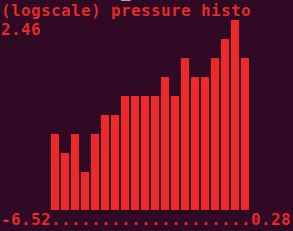printInfo()[source]

Print information about a vtk object.

rotate(angle, axis=(1, 0, 0), axis_point=(0, 0, 0), rad=False)[source]

Rotate Actor around an arbitrary axis passing through axis_point.

rotateX(angle, rad=False)[source]

Rotate around x-axis. If angle is in radians set rad=True.

NB: actor.rotateX(12).rotateY(14) will rotate FIRST around Y THEN around X.

rotateY(angle, rad=False)[source]

Rotate around y-axis. If angle is in radians set rad=True.

NB: actor.rotateX(12).rotateY(14) will rotate FIRST around Y THEN around X.

rotateZ(angle, rad=False)[source]

Rotate around z-axis. If angle is in radians set rad=True.

NB: actor.rotateX(12).rotateZ(14) will rotate FIRST around Z THEN around X.

scale(s=None)[source]

Set/get actor’s scaling factor.

Parameters: s (float, list) – scaling factor(s).

Note

if s==(sx,sy,sz) scale differently in the three coordinates.

setTransform(T)[source]

Transform object position and orientation wrt to its polygonal mesh, which remains unmodified.

show(**options)[source]

Create on the fly an instance of class Plotter or use the last existing one to show one single object.

Allowed input objects are: Actor or Volume.

This is meant as a shortcut. If more than one object needs to be visualised please use the syntax show([actor1, actor2,…], options).

Parameters: newPlotter (bool) – if set to True, a call to show will instantiate a new Plotter object (a new window) instead of reusing the first created. See e.g.: readVolumeAsIsoSurface.py the current Plotter class instance.

Note

E.g.:

from vtkplotter import *
s = Sphere()
s.show(at=1, N=2)
c = Cube()
c.show(at=0, interactive=True)

time(t=None)[source]

Set/get actor’s absolute time.

updateTrail()[source]
x(position=None)[source]

Set/Get actor position along x axis.

y(position=None)[source]

Set/Get actor position along y axis.

z(position=None)[source]

Set/Get actor position along z axis.

## Volume¶

class vtkplotter.actors.Volume(inputobj, c=('b', 'lb', 'lg', 'y', 'r'), alpha=(0.0, 0.0, 0.2, 0.4, 0.8, 1), alphaGradient=None, mode=0, origin=None, spacing=None, mapperType='gpu')[source]

Bases: vtkRenderingCorePython.vtkVolume, vtkplotter.actors.Prop

Derived class of vtkVolume. Can be initialized with a numpy object, see e.g.: numpy2volume.py

Parameters: c (float, list) – sets colors along the scalar range, or a matplotlib color map name alphas – sets transparencies along the scalar range origin (list) – set volume origin coordinates spacing (list) – voxel dimensions in x, y and z. mapperType (str) – either ‘gpu’, ‘opengl_gpu’, ‘fixed’ or ‘smart’ mode (int) – define the volumetric rendering style: 0, Composite rendering 1, maximum projection rendering 2, minimum projection 3, average projection 4, additive mode

Hint

if a list of values is used for alphas this is interpreted as a transfer function along the range of the scalar.

alpha(alpha)[source]

Assign a set of tranparencies to a volume along the range of the scalar value. A single constant value can also be assigned.

E.g.: say alpha=(0.0, 0.3, 0.9, 1) and the scalar range goes from -10 to 150. Then all voxels with a value close to -10 will be completely transparent, voxels at 1/4 of the range will get an alpha equal to 0.3 and voxels with value close to 150 will be completely opaque.

alphaGradient(alphaGrad)[source]

Assign a set of tranparencies to a volume’s gradient along the range of the scalar value. A single constant value can also be assigned. The gradient function is used to decrease the opacity in the “flat” regions of the volume while maintaining the opacity at the boundaries between material types. The gradient is measured as the amount by which the intensity changes over unit distance.

color(col)[source]

Assign a color or a set of colors to a volume along the range of the scalar value. A single constant color can also be assigned. Any matplotlib color map name is also accepted, e.g. volume.color('jet').

E.g.: say that your voxel scalar runs from -3 to 6, and you want -3 to show red and 1.5 violet and 6 green, then just set:

volume.color(['red', 'violet', 'green'])

crop(top=None, bottom=None, right=None, left=None, front=None, back=None, VOI=())[source]

Crop a Volume object.

Parameters: top (float) – fraction to crop from the top plane (positive z) bottom (float) – fraction to crop from the bottom plane (negative z) front (float) – fraction to crop from the front plane (positive y) back (float) – fraction to crop from the back plane (negative y) right (float) – fraction to crop from the right plane (positive x) left (float) – fraction to crop from the left plane (negative x) VOI (list) – extract Volume Of Interest expressed in voxel numbers Eg.: vol.crop(VOI=(xmin, xmax, ymin, ymax, zmin, zmax)) # all integers nrs
cutWithPlane(origin=(0, 0, 0), normal=(1, 0, 0))[source]

Cuts Volume with the plane defined by a point and a normal creating a tetrahedral mesh object. Makes sense only if the plane is not along any of the cartesian planes, otherwise use crop() which is way faster.

Parameters: origin – the cutting plane goes through this point normal – normal of the cutting plane
dimensions()[source]

Return the nr. of voxels in the 3 dimensions.

imagedata()[source]

Return the underlying vtkImagaData object.

isosurface(threshold=True, connectivity=False)[source]

Return an Actor isosurface extracted from the Volume object.

Parameters: threshold (float, list) – value or list of values to draw the isosurface(s) connectivity (bool) – if True only keeps the largest portion of the polydata
jittering(status=None)[source]

If jittering is True, each ray traversal direction will be perturbed slightly using a noise-texture to get rid of wood-grain effects.

legosurface(vmin=None, vmax=None, cmap='afmhot_r')[source]

Represent a Volume as lego blocks (voxels). By default colors correspond to the volume’s scalar. Returns an Actor.

Parameters: vmin (float) – the lower threshold, voxels below this value are not shown. vmax (float) – the upper threshold, voxels above this value are not shown. cmap (str) – color mapping of the scalar associated to the voxels.
mirror(axis='x')[source]

Mirror flip along one of the cartesian axes.

Note

axis='n', will flip only mesh normals.

mode(mode=None)[source]

Define the volumetric rendering style.

• 0, Composite rendering
• 1, maximum projection rendering
• 2, minimum projection
• 3, average projection
normalize()[source]

Normalize that scalar components for each point.

permuteAxes(x, y, z)[source]

Reorder the axes of the Volume by specifying the input axes which are supposed to become the new X, Y, and Z.

resize(*newdims)[source]

Increase or reduce the number of voxels of a Volume with interpolation.

scaleVoxels(scale=1)[source]

Scale the voxel content by factor scale.

threshold(vmin=None, vmax=None, replaceWith=0)[source]

Binary or continuous volume thresholding. Find the voxels that contain the value below/above or inbetween [vmin, vmax] and replaces it with the provided value (default is 0).

xSlice(i)[source]

Extract the slice at index i of volume along x-axis.

ySlice(j)[source]

Extract the slice at index j of volume along y-axis.

zSlice(k)[source]

Extract the slice at index i of volume along z-axis.

## collection¶

vtkplotter.actors.collection()[source]

Return the list of objects which have been created so far, without having to assign them a name. Useful in loops.

Example: from vtkplotter import Cone, collection, show for i in range(10): Cone(pos=[3*i, 0, 0], axis=[i, i-5, 0]) show(collection()) # in python3 you can simply use ellipses (three points symbol): show(...) 

## merge¶

vtkplotter.actors.merge(*actors)[source]

Build a new actor formed by the fusion of the polygonal meshes of the input objects. Similar to Assembly, but in this case the input objects become a single mesh.